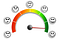# Sentiment Analysis Analysis Part 2 —Support Vector Machines

In the next set of topics we will dive into different approachs to solve the hello world problem of the NLP world, the sentiment analysis.

Check the other parts: Part1 Part2 Part3

The code for this implementation is at https://github.com/iolucas/nlpython/blob/master/blog/sentiment-analysis-analysis/svm.ipynbSentiment analysis is an area of research that aims to tell if the sentiment of a portion of text is positive or negative.

## The SVM Classifier

This classifier works trying to create a line that divides the dataset leaving the larger margin as possible between points called support vectors. As per the figure below, the line A has a larger margin than the line B, so the points divided by the line A have to travel much more to cross the division, than if the data was divided by B, so in this case we would choose the line A.

## The Code

For this task we will use scikit-learn, an open source machine learning library.

Our dataset is composed of movie reviews and labels telling whether the review is negative or positive. Let’s load the dataset:

The reviews file is a little big, so it is in zip format. Let’s Extract it:

`import zipfilewith zipfile.ZipFile("reviews.zip", 'r') as zip_ref:    zip_ref.extractall(".")`

Now that we have the reviews.txt and labels.txt files, we load them to the memory:

`with open("reviews.txt") as f:    reviews = f.read().split("\n")with open("labels.txt") as f:    labels = f.read().split("\n")    reviews_tokens = [review.split() for review in reviews]`

Next we load the module to transform our review inputs into binary vectors with the help of the class :

`from sklearn.preprocessing import MultiLabelBinarizeronehot_enc = MultiLabelBinarizer()onehot_enc.fit(reviews_tokens)`

After that we split the data into training and test set with the function:

`from sklearn.model_selection import train_test_splitX_train, X_test, y_train, y_test = train_test_split(reviews_tokens, labels, test_size=0.25, random_state=None)`

We then create our SVM classifier with the class and train it:

`from sklearn.svm import LinearSVClsvm = LinearSVC()lsvm.fit(onehot_enc.transform(X_train), y_train)`

Training the model took about 2 seconds.

After training, we use the function to check the performance of the classifier:

`score = lsvm.score(onehot_enc.transform(X_test), y_test)`

Computing the score took about 1 second only!

Running the classifier a few times we get around 85% of accuracy, basically the same of the result of the naive bayes classifier.

See ya!

Written by

## More From Medium

#### Also tagged NLP

Apr 2 · 9 min read